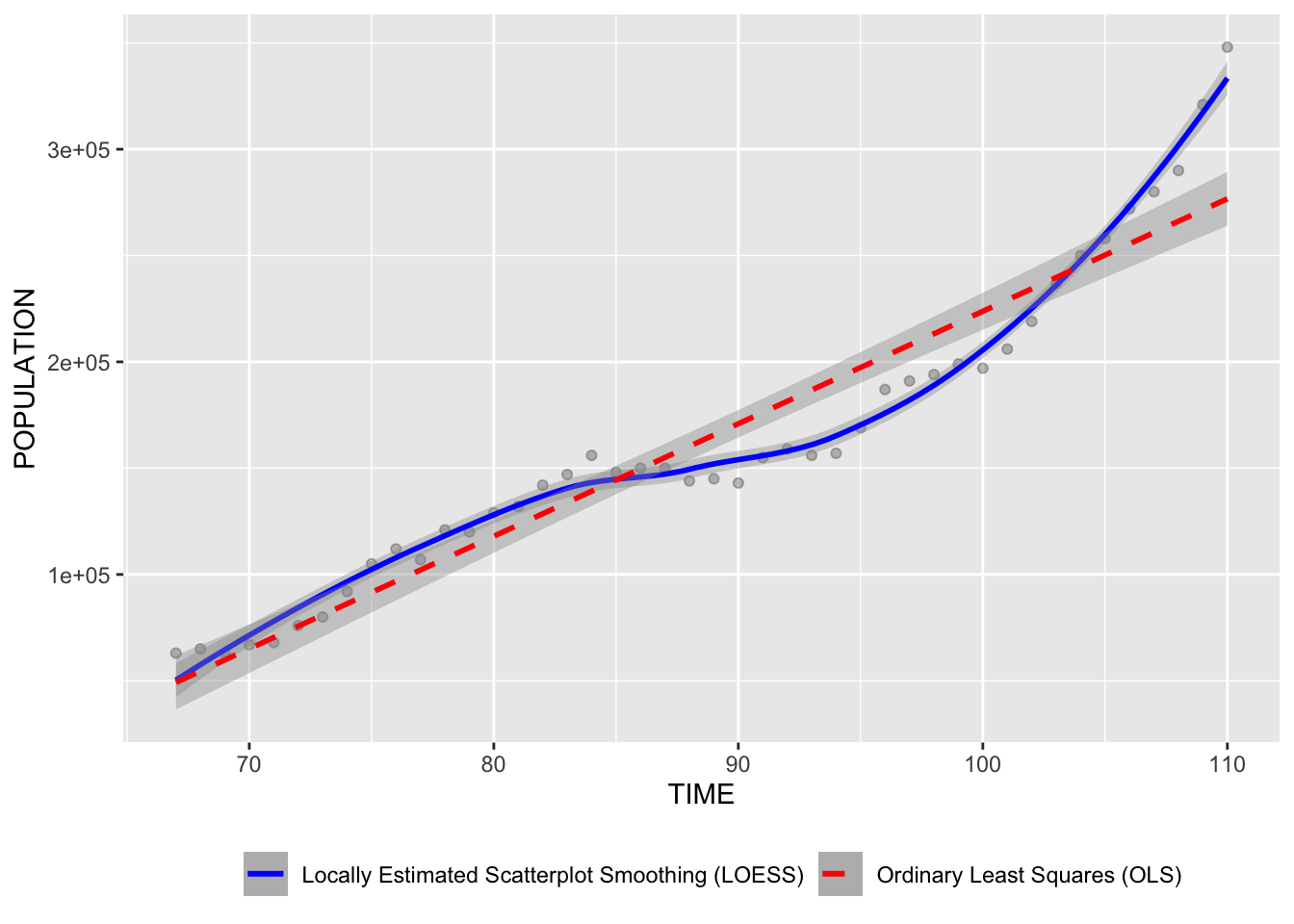Title: The elderly employment

Data Description. The data is the number of employments over 65 years old during 1978 to 2021 October. The dependent variable is population over 65 years old and the independent variable is the time. The source is from the link: https://www.stat.gov.tw/ct.asp?xItem=37135&ctNode=517&mp=4.

Discussion. LOESS 在民國85到95年之間較平緩，但整體來說，LOESS和OLS都是呈現上升的趨勢，也就是說隨著時間的過去，65歲以上的就業者也越來越多了，也呈現出了高齡化的趨勢。R Code. The code to create the above figure is shown below for reference.

# Install the rmarkdown and knitr packages
# Create a new R Markdown document with File in New File

# Install the tinytex package and run tinytex::install_tinytex() in Console to knit to pdf

library(tidyverse)

Data <- read.csv(file = "/Users/yting/Desktop/R data.csv")

ggplot(Data, aes(x = time, y = population)) +

geom_point(alpha = 0.25) +

geom_smooth(method = "loess", aes(color = "blue"), linetype = 1) +

geom_smooth(method = "lm", aes(color = "red"), linetype = 2) +

labs(x = "TIME", y = "POPULATION") +

theme(legend.position = "bottom") +

scale_color_identity(guide = "legend",

name = " ",

labels = c("Locally Estimated Scatterplot Smoothing (LOESS)",

"Ordinary Least Squares (OLS)")) +

guides(color = guide_legend(override.aes = list(linetype = c(1, 2))))